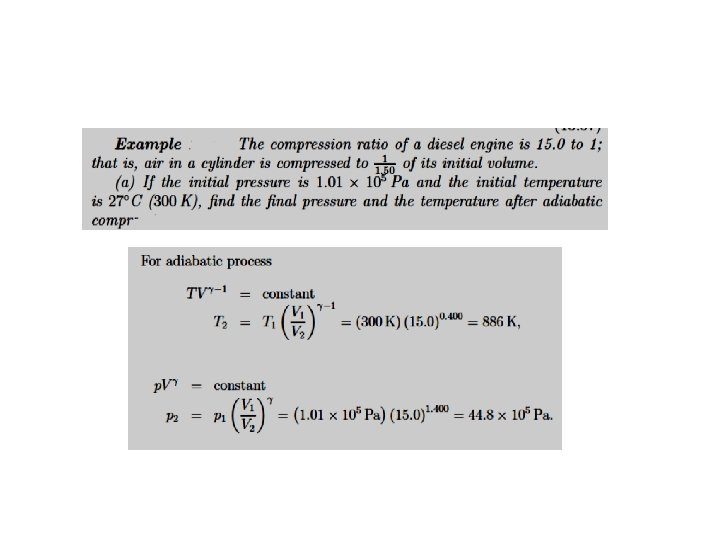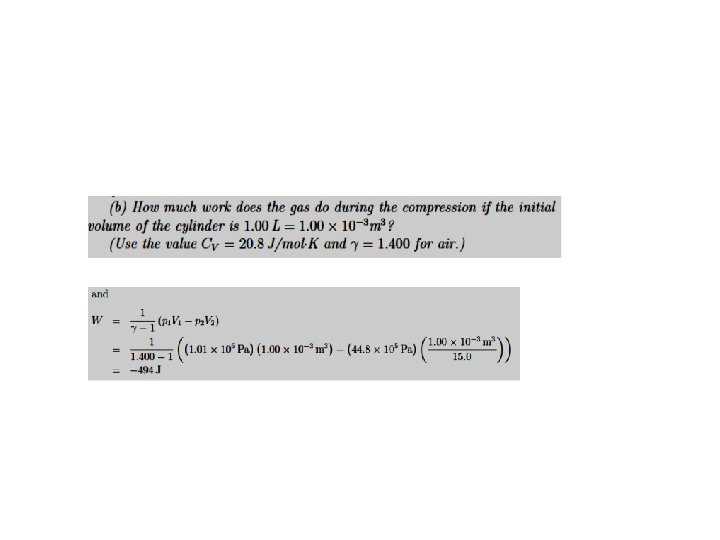Lecture one Thermodynamics The First Law of Thermodynamics

• Slides: 33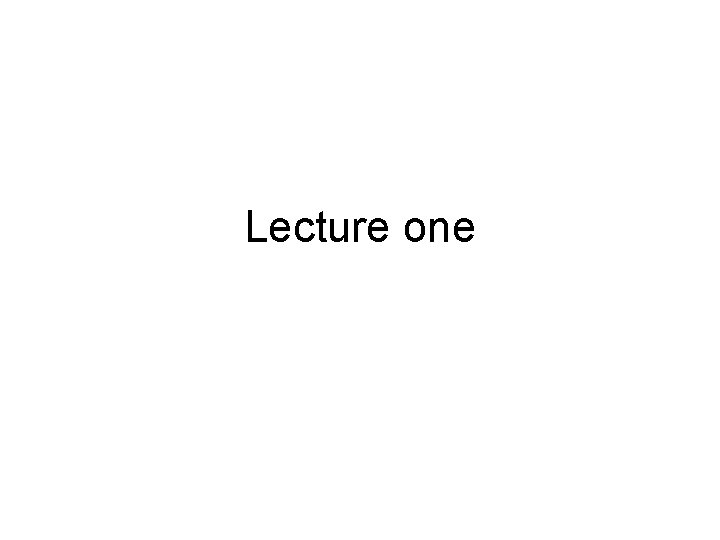Lecture one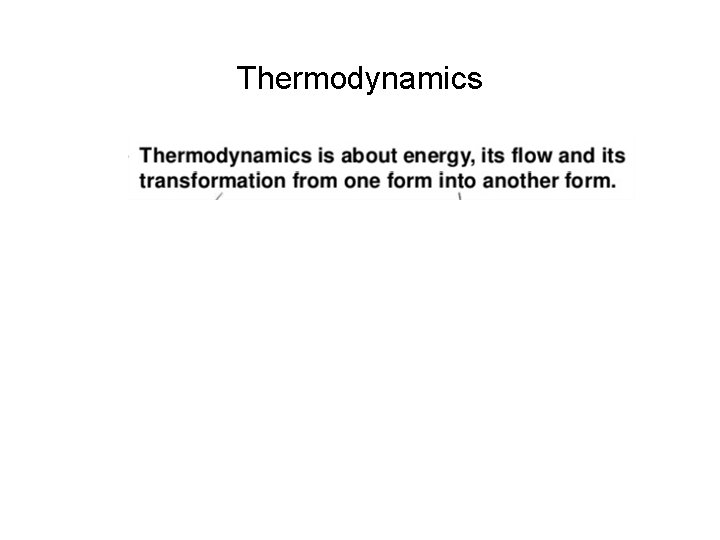Thermodynamics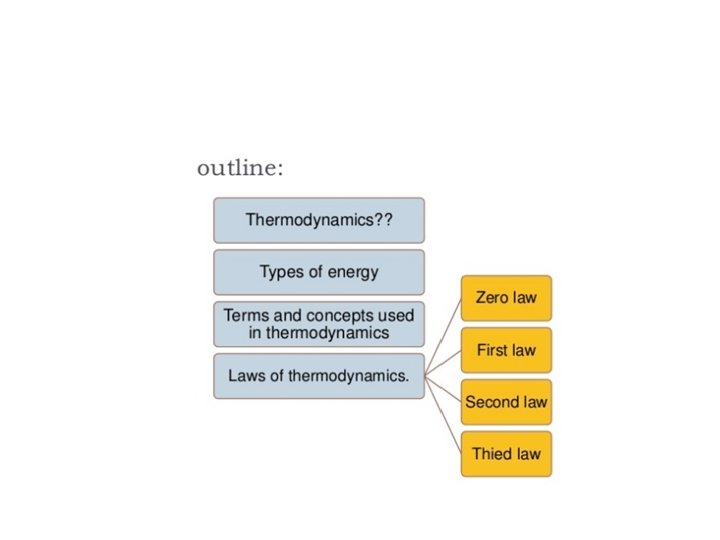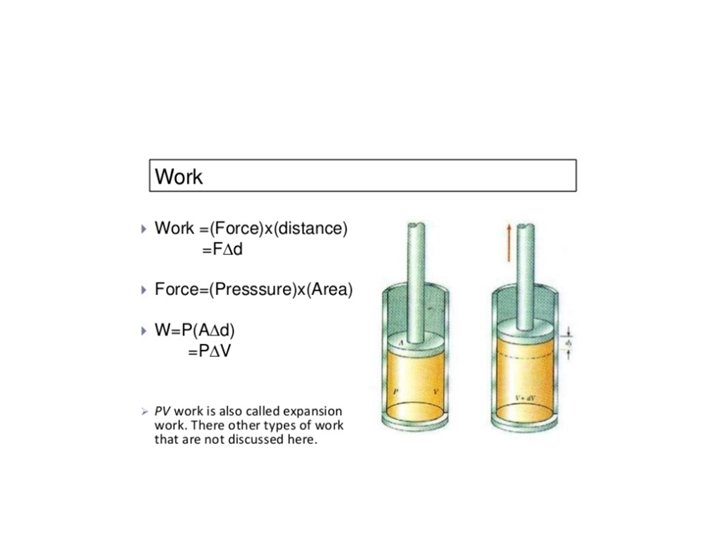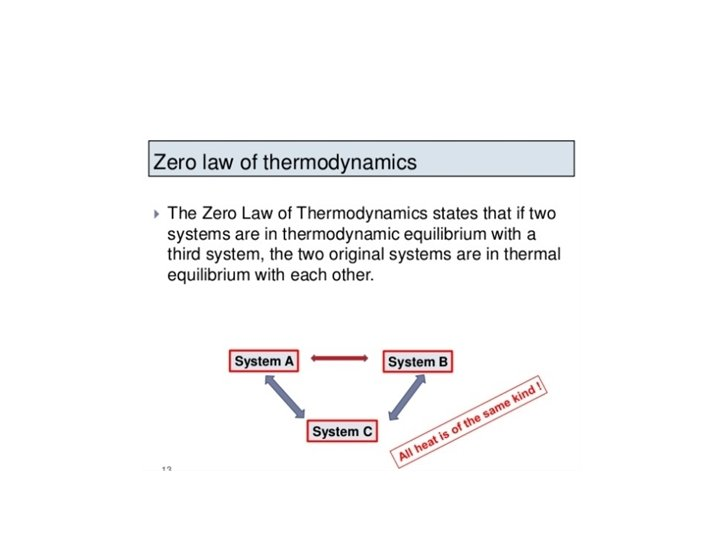The First Law of Thermodynamics Energy exists in many forms, such as mechanical energy, heat, light, chemical energy, and electrical energy. Energy is the ability to bring about change or to do work. Thermodynamics is the study of energy. Surroundings System The boundary of the system is arbitrarily chosen The system can exchange mass and energy through the boundary with the environment. An example of “closed system” - no mass flow- is the gas confined in a cylinder. The boundary –in this case real wall- is made by the cylinder and the piston walls.The First Law of Thermodynamics → Conservation of Energy: Energy can be changed from one form to another, but it cannot be created or destroyed. The total amount of energy and matter in the Universe remains constant, merely changing from one form to another. The First Law of Thermodynamics (Conservation) states that energy is always conserved, it cannot be created or destroyed. In essence, energy can be converted from one form into another. We need to define the concept of internal energy of the system, Eint as an energy stored in the system.The First Law of Thermodynamics. Heat, Work and Internal Energy Joule’s Experiment and the First Law of Thermodynamics. Equivalence between work and heat 1 calorie = 4. 184 Joules Work is done on water. The energy is transferred to the water – i. e. the system-. The energy transferred appears as an increase in temperature. We can replace the insulating walls by conducting walls. We can transfer heat through the walls to the system to produce the same increase in temperature. The increase in temperature of the system is a consequence of an increase in Internal Energy. Internal energy is a state function of the system The sum of the heat transferred into the system and the work done on the system equals the change in the internal energy of the system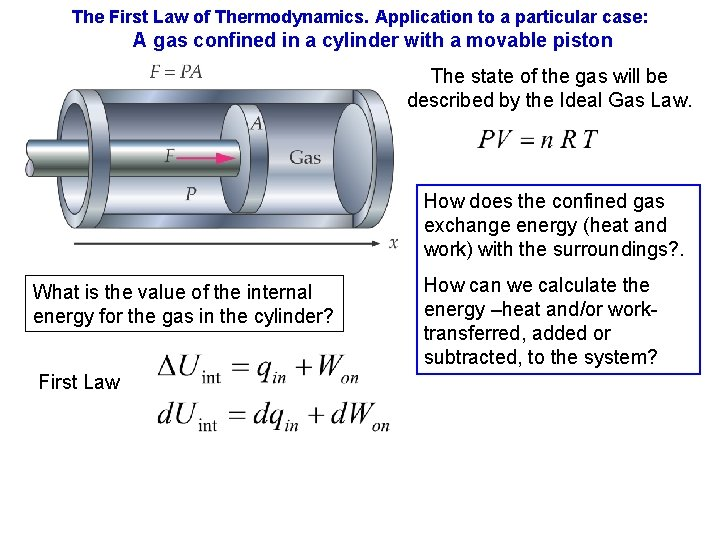The First Law of Thermodynamics. Application to a particular case: A gas confined in a cylinder with a movable piston The state of the gas will be described by the Ideal Gas Law. How does the confined gas exchange energy (heat and work) with the surroundings? . What is the value of the internal energy for the gas in the cylinder? First Law How can we calculate the energy –heat and/or worktransferred, added or subtracted, to the system?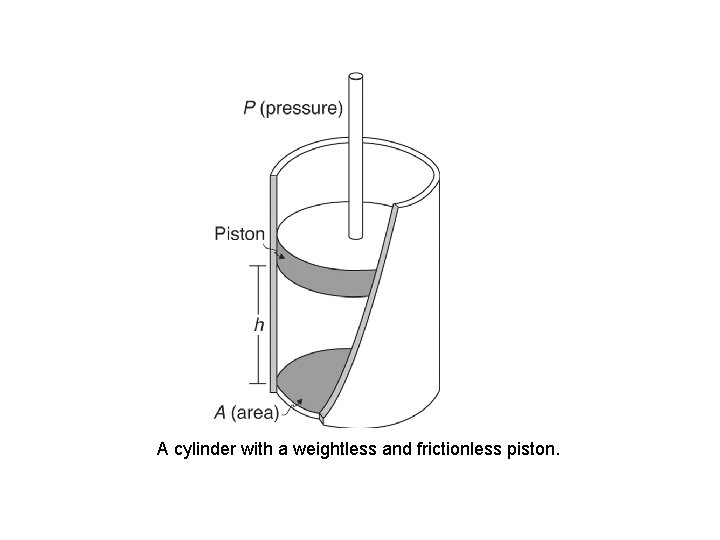A cylinder with a weightless and frictionless piston.The First Law of Thermodynamics. Application to a particular case: A gas confined in a cylinder with a movable piston Work done on the system, Won , is the energy transferred as work to the system. When this energy is added to the system its value will be positive. The work done on the gas in an expansion is P- V diagrams Constant pressure If 5 L of an ideal gas at a pressure of 2 atm is cooled so that it contracts at constant pressure until its volume is 3 L what is the work done on the gas? [405. 2 J]The First Law of Thermodynamics. P-V diagrams P- V diagrams Conecting an initial state and a final state by three paths Constant pressure Constant Volume Constant Temperature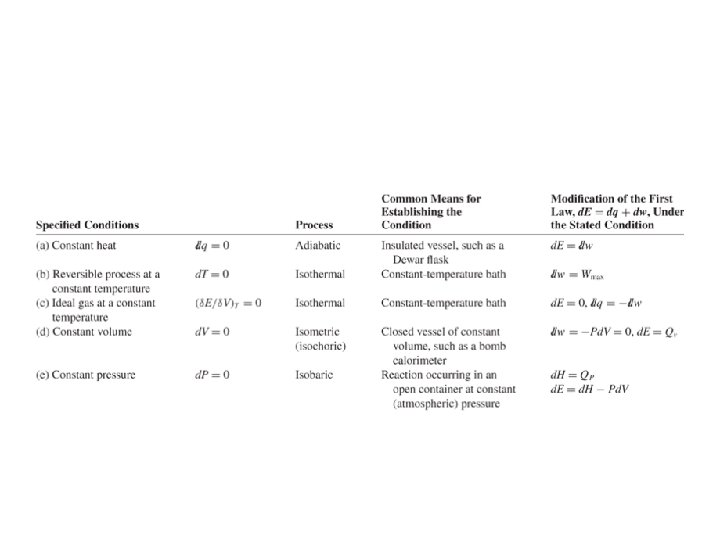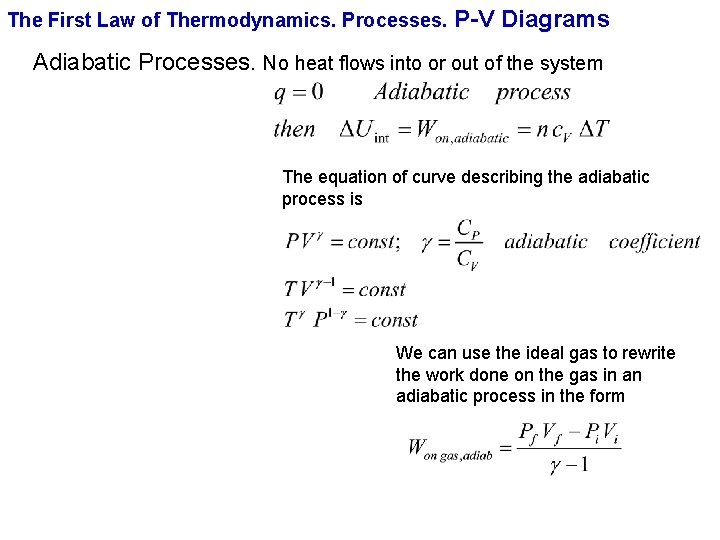The First Law of Thermodynamics. Processes. P-V Diagrams Adiabatic Processes. No heat flows into or out of the system The equation of curve describing the adiabatic process is We can use the ideal gas to rewrite the work done on the gas in an adiabatic process in the form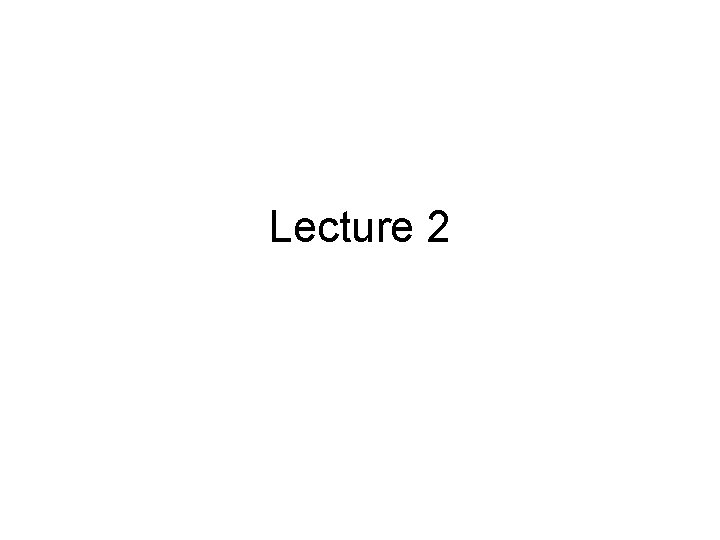Lecture 2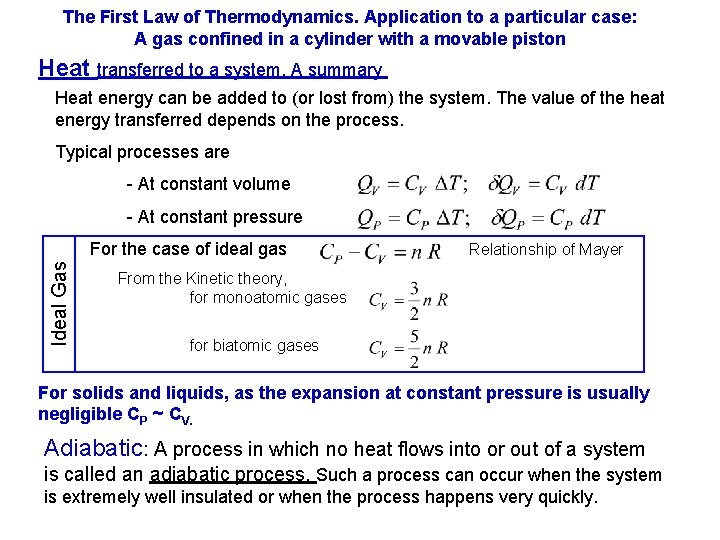The First Law of Thermodynamics. Application to a particular case: A gas confined in a cylinder with a movable piston Heat transferred to a system. A summary Heat energy can be added to (or lost from) the system. The value of the heat energy transferred depends on the process. Typical processes are - At constant volume - At constant pressure Ideal Gas For the case of ideal gas Relationship of Mayer From the Kinetic theory, for monoatomic gases for biatomic gases For solids and liquids, as the expansion at constant pressure is usually negligible CP ~ CV. Adiabatic: A process in which no heat flows into or out of a system is called an adiabatic process. Such a process can occur when the system is extremely well insulated or when the process happens very quickly.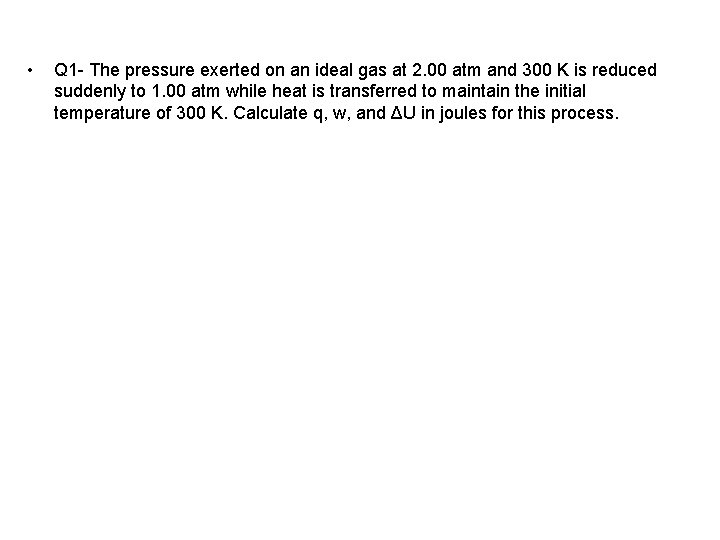• Q 1 - The pressure exerted on an ideal gas at 2. 00 atm and 300 K is reduced suddenly to 1. 00 atm while heat is transferred to maintain the initial temperature of 300 K. Calculate q, w, and ΔU in joules for this process.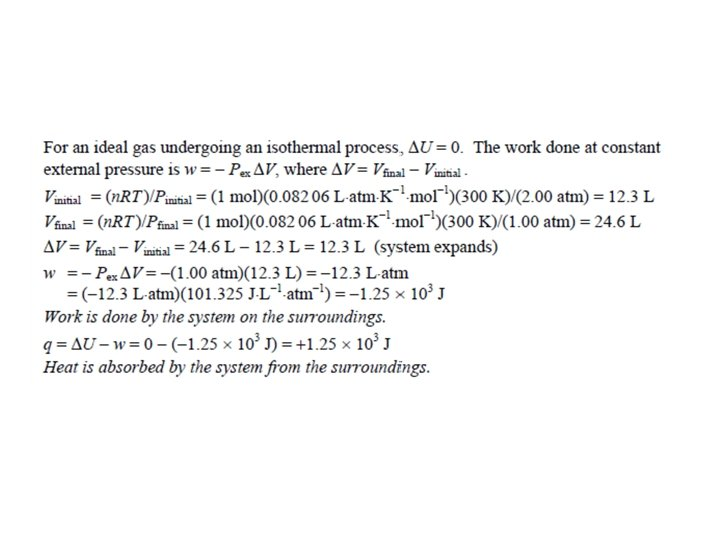• • Suppose the pressure change in previous question carried out reversibly as well as isothermally. Calculate q, w, and ΔU in joules for this process and compare the answers to those in the irreversible expansion in one step. Solution: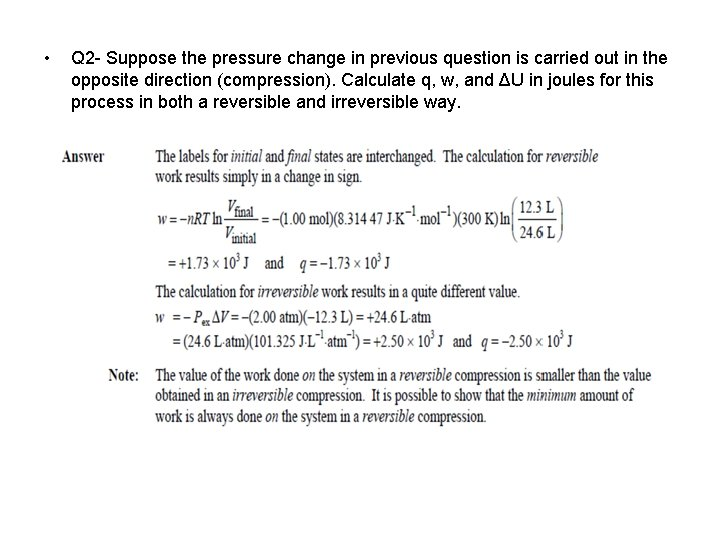• Q 2 - Suppose the pressure change in previous question is carried out in the opposite direction (compression). Calculate q, w, and ΔU in joules for this process in both a reversible and irreversible way.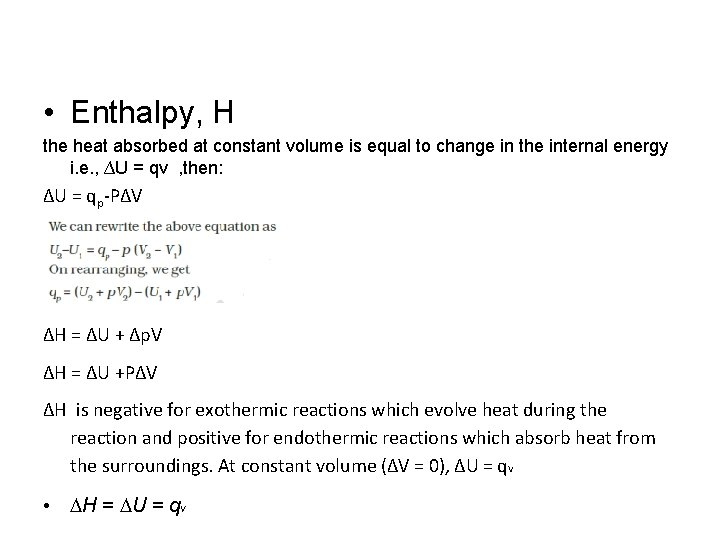• Enthalpy, H the heat absorbed at constant volume is equal to change in the internal energy i. e. , ∆U = qv , then: ∆U = qp-P∆V ∆H = ∆U + ∆p. V ∆H = ∆U +P∆V ∆H is negative for exothermic reactions which evolve heat during the reaction and positive for endothermic reactions which absorb heat from the surroundings. At constant volume (∆V = 0), ∆U = q. V • DH = DU = q V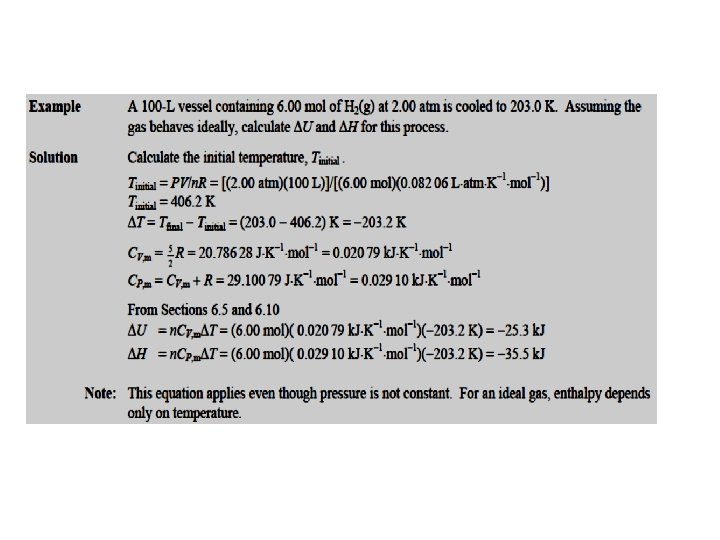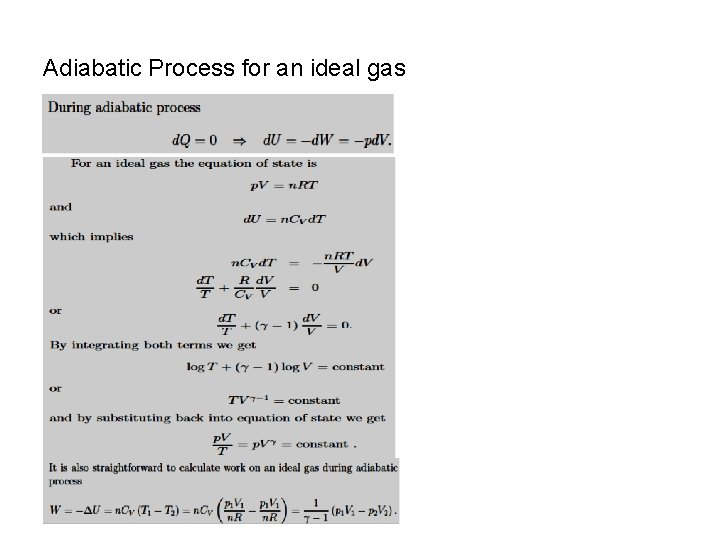Adiabatic Process for an ideal gas